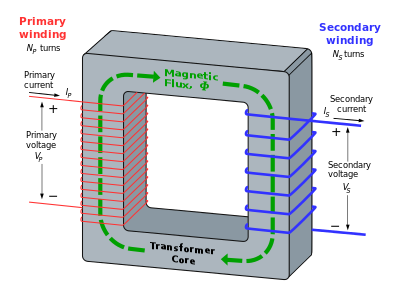# Electric transformer

Level 1The above is the diagram of an electric transformer. If the numbers of turns in the primary and secondary coil are $200$ and $100,$ respectively, and the primary voltage is $220$ V, then what is the secondary voltage?

×